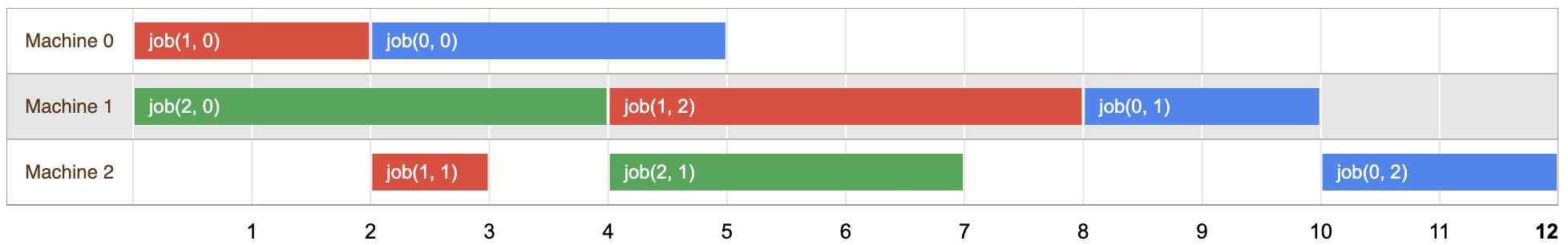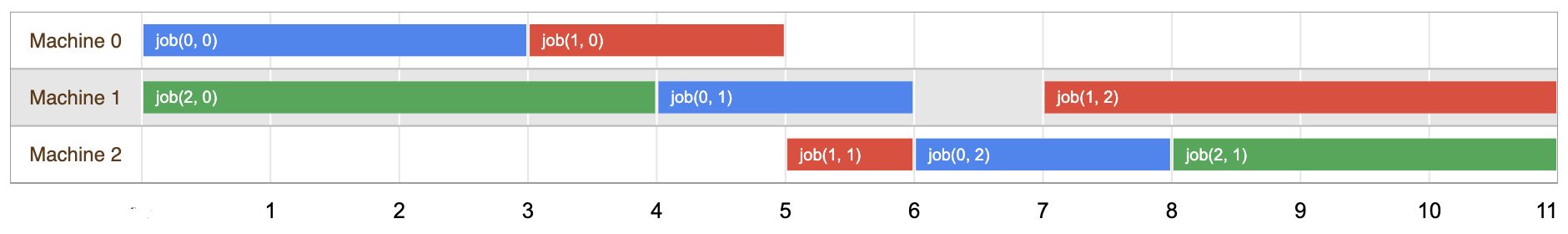# The Job Shop Problem

One common scheduling problem is the job shop, in which multiple jobs are processed on several machines. Each job consists of a sequence of tasks, which must be performed in a given order, and each task must be processed on a specific machine. For example, the job could be the manufacture of a single consumer item, such as an automobile. The problem is to schedule the tasks on the machines so as to minimize the length of the schedule—the time it takes for all the jobs to be completed.

There are several constraints for the job shop problem:

• No task for a job can be started until the previous task for that job is completed.
• A machine can only work on one task at a time.
• A task, once started, must run to completion.

## Example Problem

Below is a simple example of a job shop problem, in which each task is labeled by a pair of numbers (m, p) where m is the number of the machine the task must be processed on and p is the processing time of the task — the amount of time it requires. (The numbering of jobs and machines starts at 0.)

• job 0 = [(0, 3), (1, 2), (2, 2)]
• job 1 = [(0, 2), (2, 1), (1, 4)]
• job 2 = [(1, 4), (2, 3)]

In the example, job 0 has three tasks. The first, (0, 3), must be processed on machine 0 in 3 units of time. The second, (1, 2), must be processed on machine 1 in 2 units of time, and so on. Altogether, there are eight tasks.

### A solution for the problem

A solution to the job shop problem is an assignment of a start time for each task, which meets the constraints given above. The diagram below shows one possible solution for the problem:You can check that the tasks for each job are scheduled at non-overlapping time intervals, in the order given by the problem.

The length of this solution is 12, which is the first time when all three jobs are complete. However, as you will see below, this is not the optimal solution to the problem.

### Variables and constraints for the problem

This section describes how to set up the variables and constraints for the problem. First, let task(i, j) denote the jth task in the sequence for job i. For example, task(0, 2) denotes the second task for job 0, which corresponds to the pair (1, 2) in the problem description.

Next, define ti, j to be the start time for task(i, j). The ti, j are the variables in the job shop problem. Finding a solution involves determining values for these variables that meet the requirement of the problem.

There are two types of constraints for the job shop problem:

• Precedence constraints — These arise from the condition that for any two consecutive tasks in the same job, the first must be completed before the second can be started. For example, task(0, 2) and task(0, 3) are consecutive tasks for job 0. Since the processing time for task(0, 2) is 2, the start time for task(0, 3) must be at least 2 units of time after the start time for task 2. (Perhaps task 2 is painting a door, and it takes two hours for the paint to dry.) As a result, you get the following constraint:

t0, 2   + 2  ≤  t0, 3

• No overlap constraints — These arise from the restriction that a machine can't work on two tasks at the same time. For example, task(0, 2) and task(2, 1) are both processed on machine 1. Since their processing times are 2 and 4, respectively, one of the following constraints must hold:

t0, 2   + 2  ≤  t2, 1 (if task(0, 2) is scheduled before task(2, 1))

or

t2, 1   + 4  ≤  t0, 2 (if task(2, 1) is scheduled before task(0, 2)).

### Objective for the problem

The objective of the job shop problem is to minimize the makespan: the length of time from the earliest start time of the jobs to the latest end time.

## A Python solution

The following sections describe the main elements of a Python program that solves the job shop problem.

### Declare the model

The following code declares the model for the problem.

```# Import Python wrapper for or-tools CP-SAT solver.
from ortools.sat.python import cp_model

def MinimalJobshopSat():
"""Minimal jobshop problem."""
# Create the model.
model = cp_model.CpModel()```

### Define the data

Next, the program defines the data for the problem.

```    jobs_data = [  # task = (machine_id, processing_time).
[(0, 3), (1, 2), (2, 2)],  # Job0
[(0, 2), (2, 1), (1, 4)],  # Job1
[(1, 4), (2, 3)]  # Job2
]

machines_count = 1 + max(task for job in jobs_data for task in job)
all_machines = range(machines_count)```

### Define the variables

The following code defines the variables in the problem.

```    # Named tuple to store information about created variables.
# Named tuple to manipulate solution information.
'start job index duration')

# Creates job intervals and add to the corresponding machine lists.
machine_to_intervals = collections.defaultdict(list)

for job_id, job in enumerate(jobs_data):
suffix = '_%i_%i' % (job_id, task_id)
start_var = model.NewIntVar(0, horizon, 'start' + suffix)
end_var = model.NewIntVar(0, horizon, 'end' + suffix)
interval_var = model.NewIntervalVar(start_var, duration, end_var,
'interval' + suffix)
end=end_var,
interval=interval_var)
machine_to_intervals[machine].append(interval_var)```

For each job and task, the program uses the solver's `NewIntVar` method to create the variables:

• `start_var`: Start time of the task.
• `end_var`: End time of the task.

The upper bound for `start_var` and `end_var` is `horizon`, the sum of the processing times for all tasks in all jobs. `horizon` is sufficiently large to complete all tasks for the following reason: if you schedule the tasks in non-overlapping time intervals (a non-optimal solution), the total length of the schedule is exactly `horizon`. So the duration of the optimal solution can't be any greater than `horizon`.

Next, the program uses the `NewIntervalVar` method to create an interval variable—whose value is a variable time interval—for the task. The inputs to `NewIntervalVar` are:

• `start_var`: Variable for the start time of the task.
• `duration`: Length of the time interval for the task.
• `end_var`: Variable for the end time of the task.
• `'interval_%i_%i' % (job, task_id))`: Name for the interval variable.

In any solution, `end_var` minus `start_var` must equal `duration`.

### Define the constraints

The following code defines the constraints for the problem.

```    # Create and add disjunctive constraints.
for machine in all_machines:

# Precedences inside a job.
for job_id, job in enumerate(jobs_data):
for task_id in range(len(job) - 1):

The program uses the solver's `AddNoOverlap` method to create the no overlap constraints, which prevent tasks for the same machine from overlapping in time.

Next, the program adds the precedence constraints, which prevent consecutive tasks for the same job from overlapping in time. For each job, the line

```  model.Add(

requires the end time of a task to occur before the start time of the next task in the job.

### Define the objective

The following code defines the objective in the problem.

```    # Makespan objective.
obj_var = model.NewIntVar(0, horizon, 'makespan')
for job_id, job in enumerate(jobs_data)
])
model.Minimize(obj_var)```

The expression

```   model.AddMaxEquality(
obj_var,
[all_tasks[(job, len(jobs_data[job]) - 1)].end for job in all_jobs])```

creates a variable `obj_var` whose value is the maximum of the end times for all jobs —that is, the makespan.

### Declares the solver

The following code declares and calls the solver.

```    # Solve model.
solver = cp_model.CpSolver()
status = solver.Solve(model)```

### Display the results

The following code displays the results, including the optimal schedule and task intervals.

```        # Create one list of assigned tasks per machine.
assigned_jobs = collections.defaultdict(list)
for job_id, job in enumerate(jobs_data):
assigned_jobs[machine].append(
job=job_id,

# Create per machine output lines.
output = ''
for machine in all_machines:
# Sort by starting time.
assigned_jobs[machine].sort()
sol_line_tasks = 'Machine ' + str(machine) + ': '
sol_line = '           '

# Add spaces to output to align columns.

sol_tmp = '[%i,%i]' % (start, start + duration)
# Add spaces to output to align columns.
sol_line += '%-10s' % sol_tmp

sol_line += '\n'
output += sol_line

# Finally print the solution found.
print('Optimal Schedule Length: %i' % solver.ObjectiveValue())
print(output)```

The optimal schedule is shown below:

```Optimal Schedule Length: 11
Machine 0: job_0_0   job_1_0
[0,3]     [3,5]
Machine 1: job_2_0   job_0_1   job_1_2
[0,4]     [4,6]     [7,11]
Machine 2: job_1_1   job_0_2   job_2_1
[5,6]     [6,8]     [8,11]
```Eagle-eyed readers examining machine 1 might wonder why job_1_2 was scheduled at time 7 instead of time 6. Both are valid solutions, but remember: the objective is to minimize the makespan. Moving job_1_2 earlier wouldn't reduce the makespan, so the two solutions are equal from the solver's perspective.

### Entire program

Finally, here is the entire program for the job shop problem.

```import collections

# Import Python wrapper for or-tools CP-SAT solver.
from ortools.sat.python import cp_model

def MinimalJobshopSat():
"""Minimal jobshop problem."""
# Create the model.
model = cp_model.CpModel()

jobs_data = [  # task = (machine_id, processing_time).
[(0, 3), (1, 2), (2, 2)],  # Job0
[(0, 2), (2, 1), (1, 4)],  # Job1
[(1, 4), (2, 3)]  # Job2
]

machines_count = 1 + max(task for job in jobs_data for task in job)
all_machines = range(machines_count)

# Computes horizon dynamically as the sum of all durations.

# Named tuple to store information about created variables.
# Named tuple to manipulate solution information.
'start job index duration')

# Creates job intervals and add to the corresponding machine lists.
machine_to_intervals = collections.defaultdict(list)

for job_id, job in enumerate(jobs_data):
suffix = '_%i_%i' % (job_id, task_id)
start_var = model.NewIntVar(0, horizon, 'start' + suffix)
end_var = model.NewIntVar(0, horizon, 'end' + suffix)
interval_var = model.NewIntervalVar(start_var, duration, end_var,
'interval' + suffix)
end=end_var,
interval=interval_var)
machine_to_intervals[machine].append(interval_var)

# Create and add disjunctive constraints.
for machine in all_machines:

# Precedences inside a job.
for job_id, job in enumerate(jobs_data):
for task_id in range(len(job) - 1):

# Makespan objective.
obj_var = model.NewIntVar(0, horizon, 'makespan')
for job_id, job in enumerate(jobs_data)
])
model.Minimize(obj_var)

# Solve model.
solver = cp_model.CpSolver()
status = solver.Solve(model)

if status == cp_model.OPTIMAL:
# Create one list of assigned tasks per machine.
assigned_jobs = collections.defaultdict(list)
for job_id, job in enumerate(jobs_data):
assigned_jobs[machine].append(
job=job_id,

# Create per machine output lines.
output = ''
for machine in all_machines:
# Sort by starting time.
assigned_jobs[machine].sort()
sol_line_tasks = 'Machine ' + str(machine) + ': '
sol_line = '           '

# Add spaces to output to align columns.

sol_tmp = '[%i,%i]' % (start, start + duration)
# Add spaces to output to align columns.
sol_line += '%-10s' % sol_tmp

sol_line += '\n'